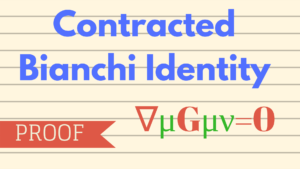# Contracted Bianchi Identity – [PROOF]The contracted Bianchi Identity in GTR can be written as:$\nabla^\mu G_{\mu \nu}=0$
where$G_{\mu \nu}$ is the Einstein Tensor such that,$G_{\mu \nu}=R_{\mu \nu}- \frac{1}{2}g_{\mu \nu}R$
and$R_{\mu \nu}$ is the Ricci Tensor and$R$ is the Ricci Scalar.

In this post, I will be demonstrating a simple way to prove the contracted Bianchi Identity.$\nabla_{\mu}R^{\quad \enspace \delta}_{\nu \lambda \rho}+\nabla_{\nu}R^{\quad \enspace \delta}_{\lambda \mu \rho}+\nabla_{\lambda}R^{\quad \enspace \delta}_{\mu \nu \rho} = 0 \qquad (\mathrm{eq. 1})$

where$R_{\nu \lambda \rho}^{\quad \enspace \delta}$ is the Riemann Tensor,
and$\nabla_{\mu}$ is the covariant derivative.

We see that there are 5 indices in the above tensors. So we go ahead and contract alternate indices twice, to get a single index tensor.

Remember the following properties of the Riemann Tensor:$R_{\mu \nu \lambda}^{\quad \enspace \rho}=-R_{\nu \mu \lambda}^{\quad \enspace \rho}$$R_{\mu \nu \lambda}^{\quad \enspace \rho}=-R_{\mu \nu \enspace \lambda}^{\enspace \enspace \rho}$$R_{\mu \nu \lambda}^{\quad \enspace \rho}=R_{\lambda \enspace \mu \nu}^{\enspace \rho}$

Now, swap (interchange) the following in the first term of$\mathrm{eq1}$:$\delta$ with$\rho$,$\nu$ with$\lambda$,
and$\enspace ^\delta_\rho$ with$\lambda \nu$$\implies \nabla_{\mu}R^{\delta}_{\enspace \rho \lambda \nu}+\nabla_{\nu}R^{\quad \enspace \delta}_{\lambda \mu \rho}+\nabla_{\lambda}R^{\quad \enspace \delta}_{\mu \nu \rho} = 0$

[Remember Riemann Tensor is pair symmetric]

Perform a similar swapping of indices, as in the last step, on the second and third term too, to get:$\implies \nabla_{\mu}R^{\delta}_{\enspace \rho \lambda \nu}+\nabla_{\nu}R^{\delta}_{\enspace \rho \mu \lambda}+\nabla_{\lambda}R^{\delta}_{\enspace \rho \nu \mu} = 0$

Operate$g^{\mu}_{\enspace \delta}=\delta^\mu_\delta$ on the above equation,

[Note that$g$ is covariantly constant and can be taken inside the covariant derivative]$g^{\mu}_{\enspace \delta}\left(\nabla_{\mu}R^{\delta}_{\enspace \rho \lambda \nu}+\nabla_{\nu}R^{\delta}_{\enspace \rho \mu \lambda}+\nabla_{\lambda}R^{\delta}_{\enspace \rho \nu \mu}\right) = 0$$\implies \nabla_{\mu}R^{\mu}_{\enspace \rho \lambda \nu}+\nabla_{\nu}R^{\mu}_{\enspace \rho \mu \lambda}+\nabla_{\lambda}R^{\mu}_{\enspace \rho \nu \mu} = 0$$\implies \nabla_{\mu}R^{\mu}_{\enspace \rho \lambda \nu}+\nabla_{\nu}R_{\rho \lambda}-\nabla_{\lambda}R^{\mu}_{\enspace \rho \mu \nu} = 0$$\implies \nabla_{\mu}R^{\mu}_{\enspace \rho \lambda \nu}+\nabla_{\nu}R_{\rho \lambda}-\nabla_{\lambda}R_{\rho \nu} = 0$

Now, operate$g^{\nu \rho}$ on the above,$\implies g^{\nu \rho}\left(\nabla_{\mu}R^{\mu}_{\enspace \rho \lambda \nu}+\nabla_{\nu}R_{\rho \lambda}-\nabla_{\lambda}R_{\rho \nu}\right) = 0$$\implies \nabla_{\mu}R^{\mu}_{\enspace \lambda}+\nabla_{\nu}R_{\enspace \lambda}^{\nu}-\nabla_{\lambda}R = 0$

Since,$\nabla_{\mu}R^{\mu}_{\enspace \lambda}=\nabla^{\mu}R_{\mu \lambda}$

Therefore,$\implies \nabla^{\mu}R_{\mu \lambda}+\nabla^{\nu}R_{\nu \lambda}-\nabla_{\lambda}R = 0$

Since,$\mu$ and$\nu$ are dummy indices, therefore, we can write$\implies 2\nabla^{\mu}R_{\mu \lambda}-\nabla_{\lambda}R = 0$$\implies 2\nabla^{\mu}R_{\mu \lambda}-g_{\mu \lambda}\nabla^{\mu}R = 0$$\implies \nabla^{\mu} \left( R_{\mu \lambda}-\frac{g_{\mu \lambda}}{2}R \right)=0$$\implies \boxed{\nabla^{\mu}G_{\mu \lambda}=0}$

Hence Proved.

### References:[wpedon id="7041" align="center"]